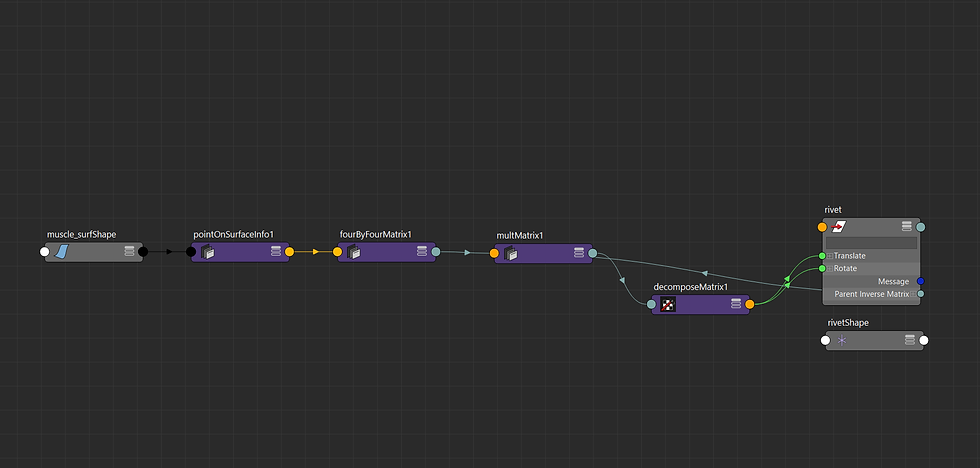top of page# Naughty Dog Muscle Recreation (Proof Of Concept)

So this is my attempt to recreate the effects of a tool made by Judd Simantov at Naughty Dog. It is a simply distance based muscle simulation.

How it works1. There are two pairs of locators.

They drive the CV position of two linear curves.

1. The are lofted to create a nurbs surface (I do this with a node but don't actually have a shape node).

2. The point on the surface (that matches the position of the midpoints of the two curves) has it's position and normal vector queried by a pointOnSurfaceInfo node.

3. The normal vector is multiplied by the bulge effect output (explained below) and added to the position.

4. Then that new position (along with the two locators positions drive the position of the CV's of a quadratic (degree 2) curve.

5. Those two resulting curves are then lofted to create a nurbs surface.

Calculating The Bulge Effect:

1. The distance between them is measured

2. The distance is divided by the global scale of the rig (to compensate for double transforms)(I did not do this in the proof of concept(

3. The distance is then divided by the original distance between the two points

4. It is then raised to the power of the bulge factor (set to -1 by default) to calculate a volume preserving effect.

5. This value is then multiplied by the starting bulge.Attaching The Locator To The Surface:

1. Get the normal and tangent-U vectors at a point on the surface and plug them into a four-by-four matrix node as the x-axis and y-axis.

2. Multiply that matrix by the parent inverse matrix of the locator (in order to cancel out the parent transforms and make the attachment work regardless of heirarchy)

3. That matrix is turned into translate and rotate attributes using a decompose matrix node.

4. Those attributes are plugged into the locator.Bringing It All Together:

You gotta love it when a node network looks so nice in the node editor.